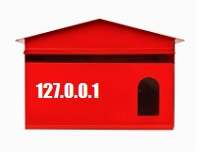# PHP function showing IP Address

This article covers the available ways to get IP address using PHP script. The ip address is the unique address for the machines like a client, server or proxy. In PHP, we can get an IP address in two ways. These are,

• By accessing the \$_SERVER global array.
• By using getenv() function.

Both of the above two methods require some key values to get the IP address of the user. We can retrieve machine address by sending the appropriate key value to any one of the above PHP controls. For example, If we want to get client IP address, then we need to call either getenv(‘HTTP_CLIENT_IP’) or \$_SERVER[‘HTTP_CLIENT_IP’].

Now, let us discuss the above two methods of showing IP address in detail.## By accessing the \$_SERVER global array.

In this method, we are attempting to access IP address as server variable that is created remotely. These variables are stored in PHP’s \$_SERVER global array.

This global array expects various key indexes to get an IP address. Those possible key indexes are listed below.

• HTTP_CLIENT_IP
• HTTP_X_FORWARDED_FOR
• HTTP_X_FORWARDED
• HTTP_FORWARDED_FOR
• HTTP_FORWARDED

Following PHP program is used to get the IP address of the user by using above possible reserved keys as the index of the \$_SERVER array.

```function showIPAddress() {
for(\$i=0;\$i<count(\$variableIndex);\$i++){
if(array_key_exists(\$variableIndex[\$i], \$_ENV)) {
break;
}
}
}
}
```

In the above PHP program, \$variableIndex array holds all possible reserved keys to find IP address. This array will be iterated to use each of its value in the \$_SERVER array until the \$ipAddress variable is set. Once a \$ipAddress variable is set, we can break the loop.

## By using getenv() function.

Instead of accessing server variables, this second method is used to get environment variable by using PHP getenv() method.

By using the same set of PHP reserved keys, IP address will be found by using getenv() function. So, in the above program, we are required to replace \$_SERVER[] with getenv() function call. Now, the program will be as shown below.

```function showIPAddress() {
for(\$i=0;\$i<count(\$variableIndex);\$i++){
if(array_key_exists(\$variableIndex[\$i], \$_SERVER)) {